Open In App

# Largest subset of Graph vertices with edges of 2 or more colors

Given an undirected complete graph with N nodes or vertices. Edges of the graph are colored, find the largest subset of vertices with edges of 2 or more colors. We are given graph as adjacency matrix C[][] where C[i][j] is color of edge from vertex i to vertex j. Since graph is undirected, values C[i][j] of C[j][i] are same.

We define C[i][i] to be zero, although there is no such edge present. i.e. the graph does not contain self-loops.

Examples:

Example 1:

Input : C[][]= {{0, 1, 2},

{1, 0, 3},

{2, 3, 0}}

Output : 3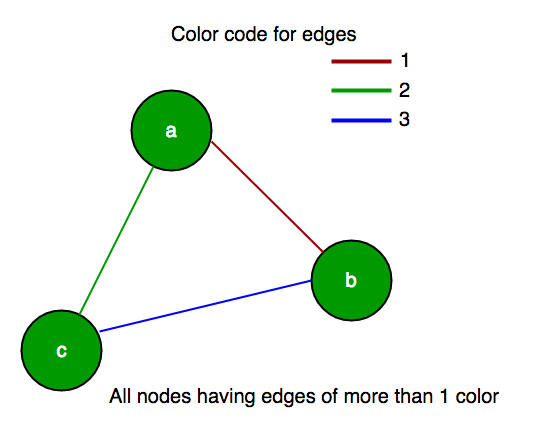Example 2:

Input  : C[][]= {{0, 1, 1},

{1, 0, 3},

{1, 3, 0}}

Output : 0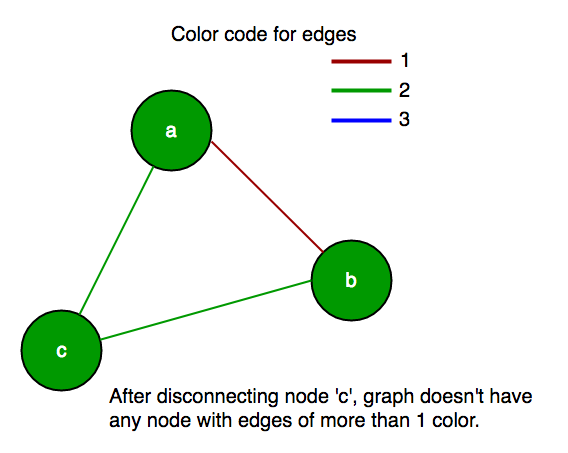Since graph is complete, each edge can be one of n*(n-1)/2 +1 different colors. These colors are labeled from 0 to n*(n-1)/2, inclusive. But not all these n*(n-1)/2 +1 colors need to be used. i.e., it is possible that two different edges could have the same color.

Let’s call a vertex “bad” if all its neighbors are of the same color. Obviously, we can’t have any such bad vertex in our subset, so remove such bad vertex from the graph. This might introduce some more bad vertices, but we can keep repeating this process until we find a subset free of bad vertices. So, at last, we should remain through a graph which does not have any bad vertex means every vertex of our subset has at least two different color edges with other adjacent vertices.

Example:
Input :
let C:
{{0, 9, 2, 4, 7, 8},
{9, 0, 9, 9, 7, 9},
{2, 9, 0, 3, 7, 6},
{4, 9, 3, 0, 7, 1},
{7, 7, 7, 7, 0, 7},
{8, 9, 6, 1, 7, 0}};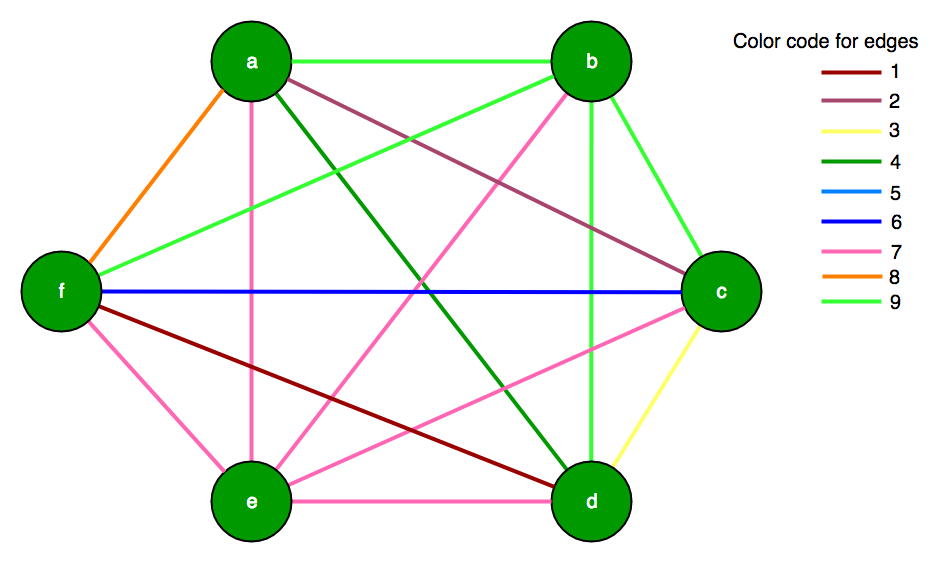Step I: First of all, we can see that row 5(node ‘e’) contains only 7 means node ‘e’ is connected through edges having color code 7 so it does not have more than one color edge so we have to remove 5 from subset. Now, our graph will contain only 5 vertex and are as:
C:
{{0, 9, 2, 4, 8},
{9, 0, 9, 9, 9},
{2, 9, 0, 3, 6},
{4, 9, 3, 0, 1},
{8, 9, 6, 1, 0}};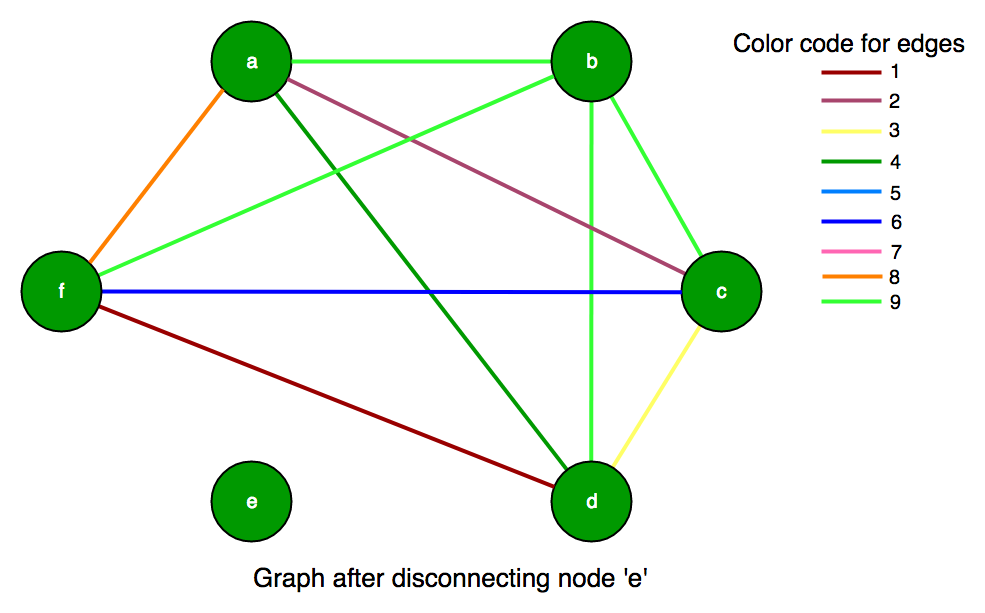Step II: Further, we can see that row 2 (node ‘b’) also doesn’t contain more than 1 color edge, so we should remove row 2 and column 2 also. Which result in our new graph as:
C:
{{0, 2, 4, 8},
{2, 0, 3, 6},
{4, 3, 0, 1},
{8, 6, 1, 0}};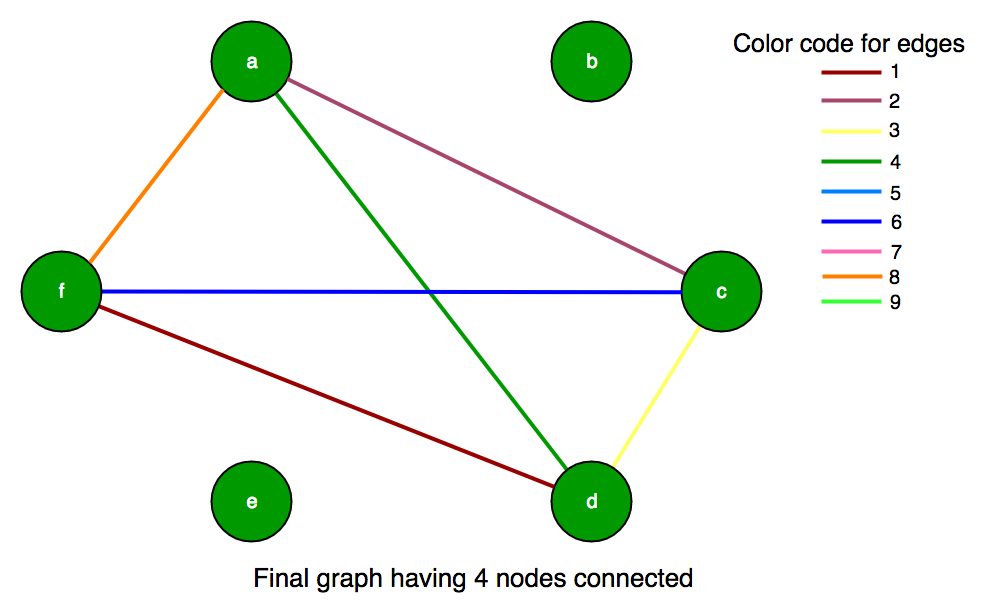Step III: Now, we can see that each vertex has more than 1 different color edge. So, the total number of vertices in the subset is 4.

Implementation:

## C++

 `// C++ program to find size of subset of graph vertex``// such that each vertex has more than 1 color edges``#include ``using` `namespace` `std;`` ` `// Number of vertices``const` `int` `N = 6;`` ` `// function to calculate max subset size``int` `subsetGraph(``int` `C[][N])``{``    ``// set for number of vertices``    ``set<``int``> vertices;``    ``for` `(``int` `i = 0; i < N; ++i)``        ``vertices.insert(i);`` ` `    ``// loop for deletion of vertex from set``    ``while` `(!vertices.empty())``    ``{``        ``// if subset has only 1 vertex return 0``        ``if` `(vertices.size() == 1)``            ``return` `1;`` ` `        ``// for each vertex iterate and keep removing``        ``// a vertex while we find a vertex with all``        ``// edges of same color.``        ``bool` `someone_removed = ``false``;``        ``for` `(``int` `x : vertices)``        ``{``            ``// note down different color values``            ``// for each vertex``            ``set<``int``> values;``            ``for` `(``int` `y : vertices)``                ``if` `(y != x)``                    ``values.insert(C[x][y]);`` ` `            ``// if only one color is found``            ``// erase that vertex (bad vertex)``            ``if` `(values.size() == 1)``            ``{``                ``vertices.erase(x);``                ``someone_removed = ``true``;``                ``break``;``            ``}``        ``}`` ` `        ``// If no vertex was removed in the``        ``// above loop.``        ``if` `(!someone_removed)``            ``break``;``    ``}`` ` `    ``return` `(vertices.size());``}`` ` `// Driver program``int` `main()``{``    ``int` `C[][N] = {{0, 9, 2, 4, 7, 8},``        ``{9, 0, 9, 9, 7, 9},``        ``{2, 9, 0, 3, 7, 6},``        ``{4, 9, 3, 0, 7, 1},``        ``{7, 7, 7, 7, 0, 7},``        ``{8, 9, 6, 1, 7, 0}``    ``};``    ``cout << subsetGraph(C);``    ``return` `0;``}`

## Java

 `// Java program to find size of ``// subset of graph vertex such that ``// each vertex has more than 1 color edges ``import` `java.util.*;`` ` `class` `GFG ``{`` ` `    ``// Number of vertices ``    ``static` `int` `N = ``6``;`` ` `    ``// function to calculate max subset size ``    ``static` `int` `subsetGraph(``int` `C[][])``    ``{``        ``// set for number of vertices ``        ``HashSet vertices = ``new` `HashSet<>();``        ``for` `(``int` `i = ``0``; i < N; ++i) ``        ``{``            ``vertices.add(i);``        ``}`` ` `        ``// loop for deletion of vertex from set ``        ``while` `(!vertices.isEmpty())``        ``{``             ` `            ``// if subset has only 1 vertex return 0 ``            ``if` `(vertices.size() == ``1``)``            ``{``                ``return` `1``;``            ``}`` ` `            ``// for each vertex iterate and keep removing ``            ``// a vertex while we find a vertex with all ``            ``// edges of same color. ``            ``boolean` `someone_removed = ``false``;``            ``for` `(``int` `x : vertices) ``            ``{``                 ` `                ``// note down different color values ``                ``// for each vertex ``                ``HashSet values = ``new` `HashSet<>();``                ``for` `(``int` `y : vertices) ``                ``{``                    ``if` `(y != x) ``                    ``{``                        ``values.add(C[x][y]);``                    ``}``                ``}`` ` `                ``// if only one color is found ``                ``// erase that vertex (bad vertex) ``                ``if` `(values.size() == ``1``) ``                ``{``                    ``vertices.remove(x);``                    ``someone_removed = ``true``;``                    ``break``;``                ``}``            ``}`` ` `            ``// If no vertex was removed in the ``            ``// above loop. ``            ``if` `(!someone_removed) ``            ``{``                ``break``;``            ``}``        ``}`` ` `        ``return` `(vertices.size());``    ``}`` ` `    ``// Driver code ``    ``public` `static` `void` `main(String[] args)``    ``{``        ``int` `C[][] = {{``0``, ``9``, ``2``, ``4``, ``7``, ``8``},``        ``{``9``, ``0``, ``9``, ``9``, ``7``, ``9``},``        ``{``2``, ``9``, ``0``, ``3``, ``7``, ``6``},``        ``{``4``, ``9``, ``3``, ``0``, ``7``, ``1``},``        ``{``7``, ``7``, ``7``, ``7``, ``0``, ``7``},``        ``{``8``, ``9``, ``6``, ``1``, ``7``, ``0``}``        ``};``        ``System.out.println(subsetGraph(C));``    ``}``} `` ` `// This code has been contributed by 29AjayKumar`

## Python3

 `# Python3 program to find size of subset ``# of graph vertex such that each vertex``# has more than 1 color edges`` ` `# function to calculate max subset size ``def` `subsetGraph(C):``    ``global` `N``     ` `    ``# set for number of vertices ``    ``vertices ``=` `set``()``    ``for` `i ``in` `range``(N):``        ``vertices.add(i) `` ` `    ``# loop for deletion of vertex from set ``    ``while` `(``len``(vertices) !``=` `0``):``         ` `        ``# if subset has only 1 vertex return 0 ``        ``if` `(``len``(vertices) ``=``=` `1``): ``            ``return` `1`` ` `        ``# for each vertex iterate and keep removing ``        ``# a vertex while we find a vertex with all ``        ``# edges of same color. ``        ``someone_removed ``=` `False``        ``for` `x ``in` `vertices:``             ` `            ``# note down different color values ``            ``# for each vertex ``            ``values ``=` `set``()``            ``for` `y ``in` `vertices:``                ``if` `(y !``=` `x): ``                    ``values.add(C[x][y]) `` ` `            ``# if only one color is found ``            ``# erase that vertex (bad vertex) ``            ``if` `(``len``(values) ``=``=` `1``):``                ``vertices.remove(x) ``                ``someone_removed ``=` `True``                ``break`` ` `        ``# If no vertex was removed in the ``        ``# above loop. ``        ``if` `(``not` `someone_removed): ``            ``break`` ` `    ``return` `len``(vertices)`` ` `# Driver Code`` ` `# Number of vertices ``N ``=` `6``C ``=` `[[``0``, ``9``, ``2``, ``4``, ``7``, ``8``], ``     ``[``9``, ``0``, ``9``, ``9``, ``7``, ``9``],``     ``[``2``, ``9``, ``0``, ``3``, ``7``, ``6``],``     ``[``4``, ``9``, ``3``, ``0``, ``7``, ``1``],``     ``[``7``, ``7``, ``7``, ``7``, ``0``, ``7``],``     ``[``8``, ``9``, ``6``, ``1``, ``7``, ``0``]]``print``(subsetGraph(C))`` ` `# This code is contributed by PranchalK`

## C#

 `// C# program to find size of ``// subset of graph vertex such that ``// each vertex has more than 1 color edges ``using` `System;``using` `System.Collections.Generic; `` ` `class` `GFG ``{`` ` `    ``// Number of vertices ``    ``static` `int` `N = 6;`` ` `    ``// function to calculate max subset size ``    ``static` `int` `subsetGraph(``int` `[,]C)``    ``{``        ``// set for number of vertices ``        ``HashSet<``int``> vertices = ``new` `HashSet<``int``>();``        ``for` `(``int` `i = 0; i < N; ++i) ``        ``{``            ``vertices.Add(i);``        ``}`` ` `        ``// loop for deletion of vertex from set ``        ``while` `(vertices.Count != 0)``        ``{``             ` `            ``// if subset has only 1 vertex return 0 ``            ``if` `(vertices.Count == 1)``            ``{``                ``return` `1;``            ``}`` ` `            ``// for each vertex iterate and keep removing ``            ``// a vertex while we find a vertex with all ``            ``// edges of same color. ``            ``Boolean someone_removed = ``false``;``            ``foreach` `(``int` `x ``in` `vertices) ``            ``{``                 ` `                ``// note down different color values ``                ``// for each vertex ``                ``HashSet<``int``> values = ``new` `HashSet<``int``>();``                ``foreach` `(``int` `y ``in` `vertices) ``                ``{``                    ``if` `(y != x) ``                    ``{``                        ``values.Add(C[x, y]);``                    ``}``                ``}`` ` `                ``// if only one color is found ``                ``// erase that vertex (bad vertex) ``                ``if` `(values.Count == 1) ``                ``{``                    ``vertices.Remove(x);``                    ``someone_removed = ``true``;``                    ``break``;``                ``}``            ``}`` ` `            ``// If no vertex was removed in the ``            ``// above loop. ``            ``if` `(!someone_removed) ``            ``{``                ``break``;``            ``}``        ``}`` ` `        ``return` `(vertices.Count);``    ``}`` ` `    ``// Driver code ``    ``public` `static` `void` `Main(String[] args)``    ``{``        ``int` `[,]C = {{0, 9, 2, 4, 7, 8},``                    ``{9, 0, 9, 9, 7, 9},``                    ``{2, 9, 0, 3, 7, 6},``                    ``{4, 9, 3, 0, 7, 1},``                    ``{7, 7, 7, 7, 0, 7},``                    ``{8, 9, 6, 1, 7, 0}};``        ``Console.WriteLine(subsetGraph(C));``    ``}``}`` ` `// This code is contributed by Rajput-Ji`

## Javascript

 ``

Output

`4`